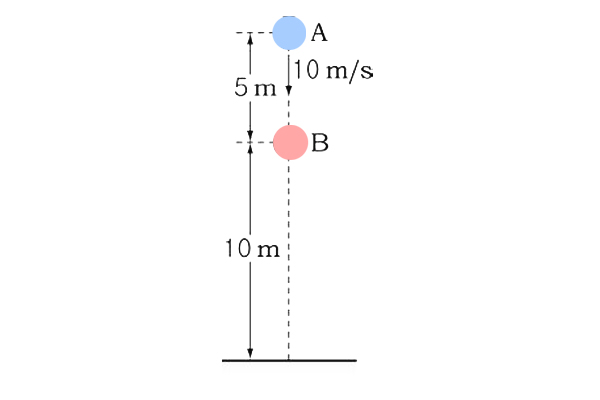# First collisionThere are two objects $A$ ($15 \text{ m}$ above ground) and $B$ ($10 \text{ m}$ above ground) on a straight line perpendicular to the ground. $A$ is thrown straight downward with a speed of $10 \text{ m/s}$ and, at the same time, $B$ starts to fall freely. Find the time that passes until their first collision.

×

Problem Loading...

Note Loading...

Set Loading...